# TDIST ExcelArticle byExcelMojo Team## What Is TDIST Function In Excel?

The TDIST function in Excel determines the Student’s t-distribution probability percentage. Users can apply this function while performing hypothesis testing of small sample datasets.

However, the TDIST Excel function got replaced in Excel 2010 with newer functions, such as T.DIST.RT and T.DIST.2T, with improved accuracy. So, it is now available as a Compatibility function for backward compatibility.

For example, the first table shows the input values required to calculate the Student’s t-distribution probability percentage.

Suppose the requirement is to determine the one- and two-tailed t-distribution probability percentages for the given input value sets and display the output in cell range D8:D9. And the target cell range D8:D9 have the Number Format set as Percentage in the Home tab.

Then applying the TDIST Excel function in the target cells will get us the required probability values.

In this TDIST Excel function example, the cell D8 formula accepts the argument values in Value Set 1. And as the third argument value is 1, the function returns the one-tailed t-distribution probability percentage, 2.55%.

Similarly, the cell D9 formula accepts the argument values in Value Set 2. And as the third argument value is 2, the function returns the two-tailed t-distribution probability percentage, 5.09%.

###### Key Takeaways
• The TDIST Excel function computes the t-distribution probability of an event across a sample data set. The function is useful for performing hypothesis testing of small sample sets.
• The TDIST function is a Compatibility Excel function, as it got replaced by newer functions, such as T.DIST.RT and T.DIST.2T, with improved accuracy.
• As input, the TDIST function accepts three mandatory arguments, x, deg_freedom, and tails.
• If x is negative, the formula you must apply to get the one-tailed t-distribution probability is 1 – TDIST(x,deg_freedom,1). And to calculate the two-tailed t-distribution probability, use the formula TDIST(x,deg_freedom,2).

### TDIST() Excel Formula

The TDIST Excel function syntax is:

where,

• x: A computed numeric value of t with which we calculate the Student’s t-distribution.
• deg_freedom: An integer value denoting the number of degrees of freedom.
• tails: The number of distribution tails you require the TDIST Excel function to return. This argument can take the value 1 or 2, indicating one- or two-tailed t-distribution.

All three arguments in the above TDIST Excel function syntax are mandatory.

Further, below are the critical aspects of the TDIST() you must know before applying the function.

• The deg_freedom and tails argument values get truncated to integer values.
• If the tails argument value is 1, the TDIST Excel function output will be P, with X, a random variable following the t-distribution, greater than x. And if the tails argument value is 2, the TDIST() output will be P, with X greater than x or less than -x.
• If x is less than 0, the TDIST(-x,deg_freedom,1) is equal to the formula 1 – TDIST(x,deg_freedom,1) and results in P, with X greater than -x. And the TDIST(-x,deg_freedom,2) is equal to TDIST(x,deg_freedom,2) and results in P, with the absolute value of X greater than x.
• If we supply a non-numeric argument value, the TDIST Excel function throws the #VALUE! error.
• Suppose the x value is less than 0, the deg_freedom value is less than 1, or the tails argument value is not 1 or 2. Then, the TDIST() output is the #NUM! error.

### How To Use TDIST Excel Function?

The steps to apply the TDIST Excelfunction are as follows:

1. First, ensure the source data contains the required numeric values and is valid.
2. Then, select the required target cell, and enter the TDIST Excel function.
3. Finally, press Enter to view the t-distribution probability percentage.

The below example explains the above steps to apply the TDIST Excel function.

The following table contains the values required to calculate the one-tailed t-distribution probability percentage.

And suppose the requirement is to determine and display the one-tailed t-distribution probability percentage in cell B8, assuming the cell’s data format is Percentage. Then, you can use the TDIST Excel function in the target cell and achieve the required probability value.

1. Select the target cell B8, enter the following TDIST(), and press Enter.

=TDIST(C3,C4,C5)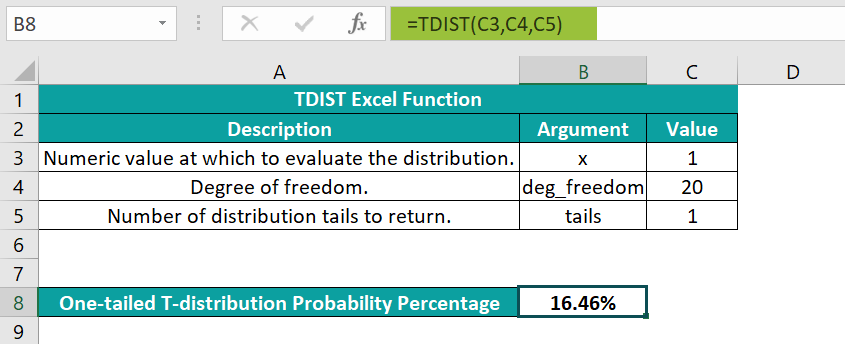You can also enter the argument values directly as numeric values, as shown below.

Once you enter the first two argument values and a comma, Excel will show the drop-down list to select the tails argument value.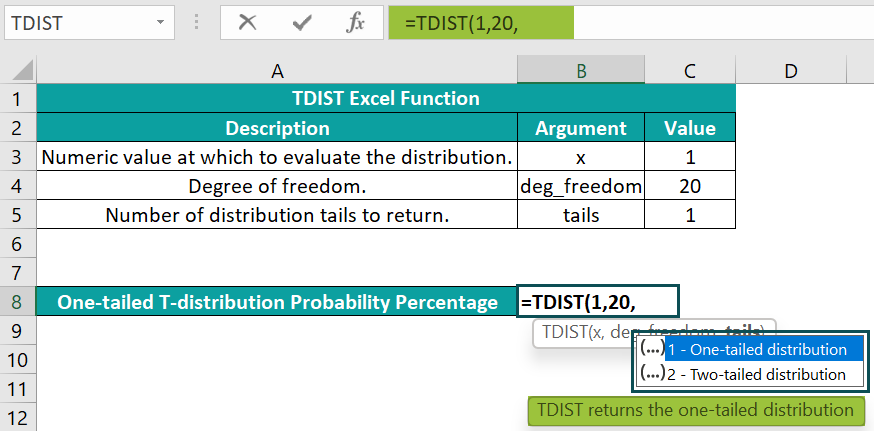The requirement is to calculate one-tailed distribution. So, double-click on the first option and close the parenthesis.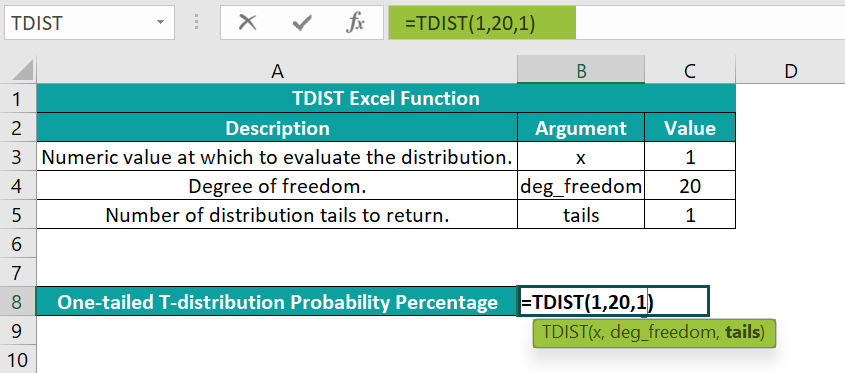And once we press Enter, the function gets executed.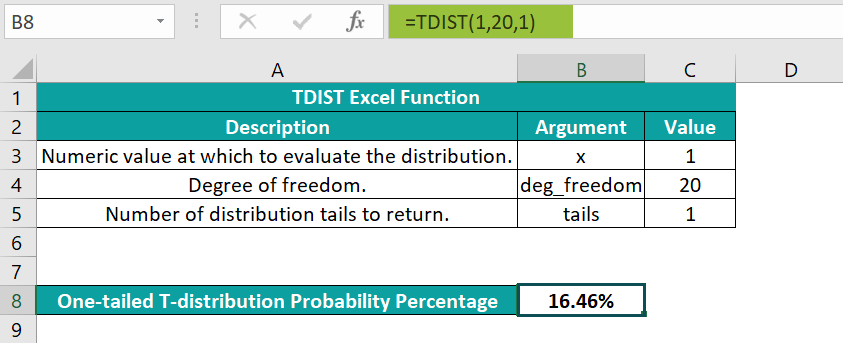Alternatively, we can enter the TDIST Excel function using the option in the Formulas tab. And for that, we must select the target cell B8 and click FormulasMore FunctionsCompatibilityTDIST to open the Function Arguments window.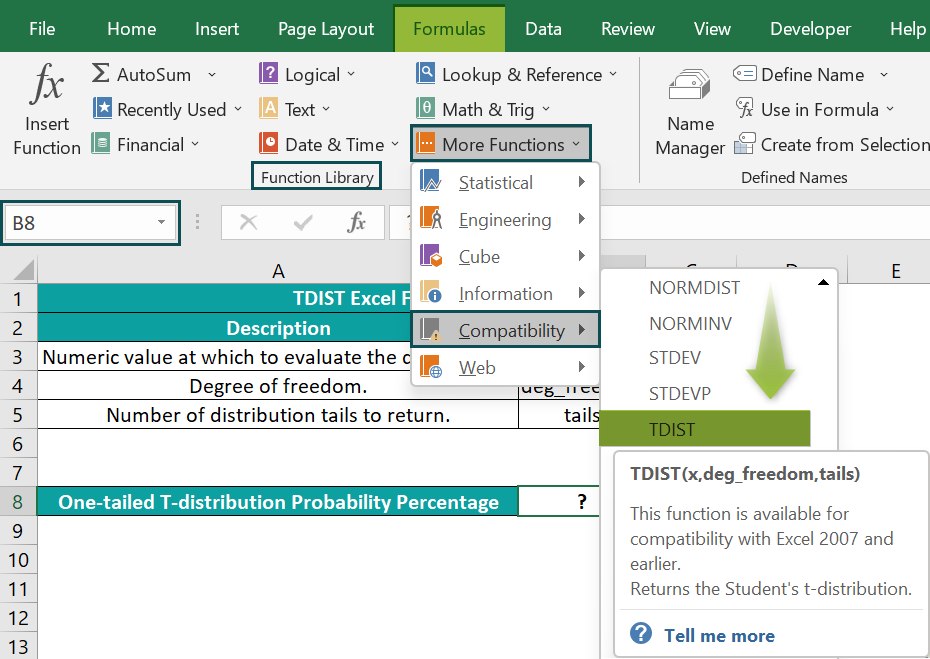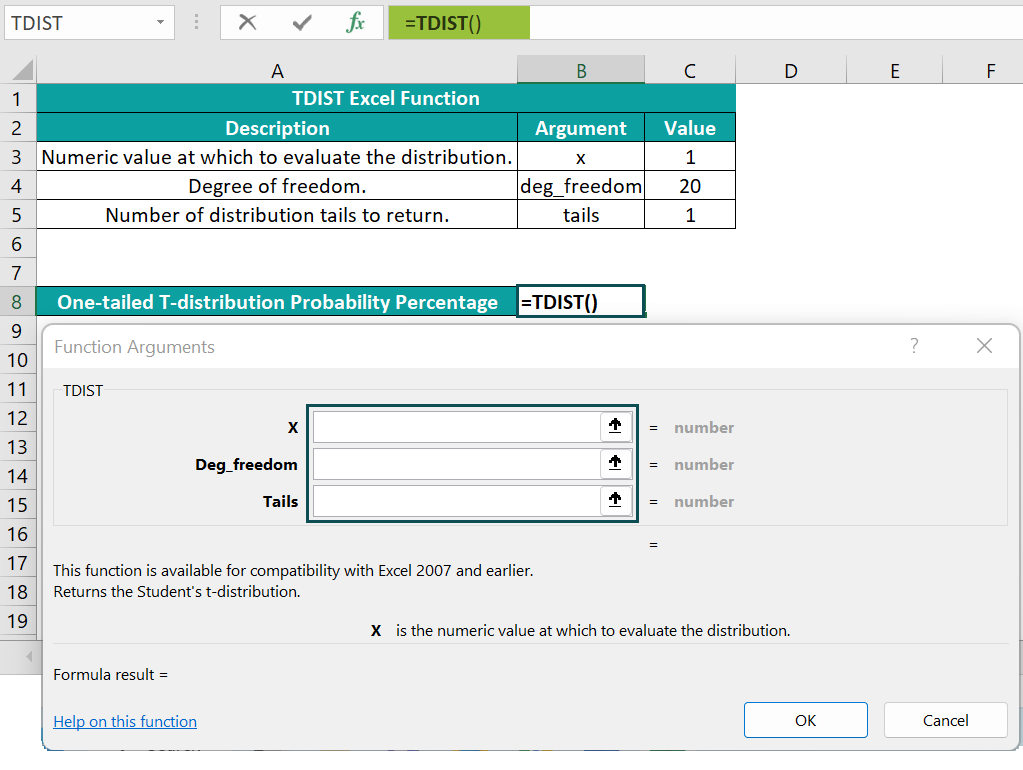In the Function Arguments window, we can enter the cell references to the respective argument values or the numbers directly in the corresponding fields.And once we click OK in the Function Arguments window, the TDIST Excel function will get executed in the target cell B8.

In this example, the one-tailed t-distribution probability for exact 1 and the given degree of freedom, 20, is 16.46%. The TDIST Excel function returns a decimal value. But, as the Number Format of the target cell is Percentage, we get the result as a percentage value.

### Examples

Check out the below TDIST Excel function examples to learn about the function effectively.

#### Example #1

We can apply the TDIST Excel function to determine the p-value in a T-Test to confirm whether to reject or go with the null hypothesis.

Consider the following table containing T-Test data.

Suppose we must determine the p-value in cell B7, based on the given date, to compare it with Alpha and decide on the null hypothesis. Then, we can apply the TDIST Excel function in the target cell and get the required p-value.

• Step 1: Select the target cell B7, enter the following TDIST Excel function, and press Enter.

=TDIST(B5,B3-1,1)

The function considers the upper end of the T-Test curve, as x can only be a positive value. And hence, we shall consider this case as one-tailed.

The TDIST() accepts the calculated t-statistic value as x. The deg_freedom value is one less than the given sample size, so the second argument takes the value 9. And the function sets the tails argument value as 1 to return the p-value as a decimal, 0.004615.

So, as the p-value is less than the Alpha, you should reject the null hypothesis.

#### Example #2

This example shows how to use the TDIST Excel function when x is negative.

The table below contains two sets of TDIST Excel function argument values, with the x value being negative in both scenarios.

Suppose the requirement is to calculate and display the one- and two-tailed t-distribution probability percentages in cell range D8:D9 for the two given value sets. And assume the target cells data format is Percentage.

Then applying the TDIST Excel function in the target cells in the below ways can fetch you the required data.

• Step 1: Select the target cell D8, enter the below formula, and press Enter.

=1-TDIST(2,60,1)

As x is negative, we need to apply the TDIST Excel function, as explained above, to obtain the one-tailed t-distribution probability percentage.

• Step 2: Select the target cell D9, enter the below formula, and press Enter.

=TDIST(2,60,2)

As x is negative, we need to apply the TDIST Excel function in the above manner when the tails argument value is 2.

#### Example #3

We shall now see how to apply the newer Excel functions, such as T.DIST, T.DIST.RT, and T.DIST.2T, which replaced the TDIST Excel function.

We can either directly enter them in the required cell or access them by clicking FormulasMore FunctionsStatistical. And they work with improved accuracy, with their names better indicating their purpose.

The first table shows the data required to supply as input to the TDIST and the latest functions.

Suppose the second table contains the different T-Test types, and the requirement is to determine the p-value in each case and display the output in cell range C9:C14.

Then, here is how we can apply the functions mentioned in the range B9:B14 in the target cells and achieve the required p-value for each T-Test type.

• Step 1: Select the target cell range C9:C14 and set the Number Format in the Home tab as Percentage to view the p-values as percentages.
• Step 2: Select the target cell C9, enter the TDIST(), and press Enter.

=TDIST(C3,C4,1)

As the T-Test type is one-tailed, the tails argument value is 1. So, the one-tailed t-distribution probability for exact 0.8 and the given degree of freedom, 5, is 23%.

• Step 3: Select the target cell C10, enter the TDIST(), and press Enter.

=TDIST(C3,C4,2)

As the T-Test type is two-tailed, the tails argument value is 2. So, the two-tailed t-distribution probability for exact 0.8 and the given degree of freedom, 5, is 46%.

• Step 4: Select the target cell C11, enter the T.DIST.RT(), and press Enter.

=T.DIST.RT(C3,C4)

As the T-Test type is right-tailed, we apply the function T.DIST.RT for better accuracy. And it accepts two argument values as input. So, the right-tailed t-distribution probability for exact 0.8 and the given degree of freedom, 5, is 23%.

• Step 5: Select the target cell C12, enter the T.DIST.2T(), and press Enter.

=T.DIST.2T(C3,C4)

As the T-Test type is two-tailed, we apply the T.DIST.2T() for better accuracy. And it accepts two argument values as input. So, the two-tailed t-distribution probability for exact 0.8 and the given degree of freedom, 5, is 46%.

• Step 6: Select the target cell C13, enter the T.DIST(), and press Enter.

=T.DIST(C3,C4,TRUE)

As the T-Test type is left-tailed and cumulative distribution, we apply the T.DIST(). It accepts the two given values as the first and second arguments and takes the third argument value as TRUE. So, the required t-distribution probability for exact 0.8 and the given degree of freedom, 5, is 77%.

• Step 7: Select the target cell C14, enter the T.DIST(), and press Enter.

=T.DIST(C3,C4,FALSE)

As the T-Test type is left-tailed and has probability density, we apply the T.DIST(). It accepts the two given values as the first and second arguments and takes the third argument value as FALSE. So, the required t-distribution probability for exact 0.8 and the given degree of freedom, 5, is 26.45%.

### Important Things To Note

• The TDIST Excel function syntax is =TDIST(x,deg_freedom,tails).
• x denotes the computed numeric value of t with which we calculate the Student’s t-distribution.
• deg_freedom is the integer value denoting the number of degrees of freedom.
• Tails shows the number of distribution tails we require the TDIST Excel function to return. It can take the value 1 or 2.
• The deg_freedom and tails get truncated to integers.
• For non-numeric argument values, the TDIST Excel function output will be the #VALUE! error.
• For x less than 0, deg_freedom less than 1, or tails not being 1 or 2, the TDIST() throws the #NUM! error.

1. How can you apply the TDIST function in Excel VBA?

You can apply the TDIST function in Excel VBA using the method:

Application.WorksheetFunction.TDist(Arg1,Arg2,Arg3)

Arguments Arg1, Arg2, and Arg3 correspond to the TDIST() arguments, x, deg_freedom, and tails.

Using an example, let us see how to apply the above method in Excel VBA.
The table below contains the TDIST() argument values.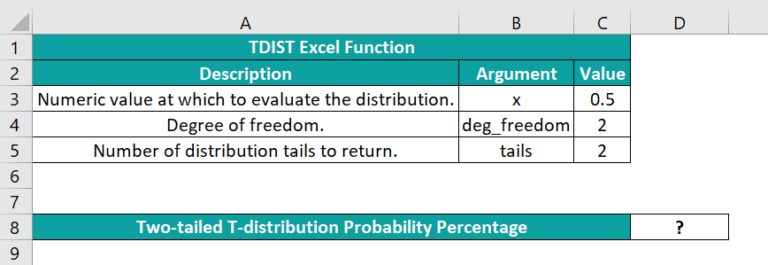And suppose the requirement is to calculate and show the two-tailed t-distribution probability percentage in cell D8 based on the given input data. Then, here is how you can apply the TDIST function in Excel VBA to display the result in the target cell.

• Step 1: Using the keys Alt + F11, open the VBA Editor.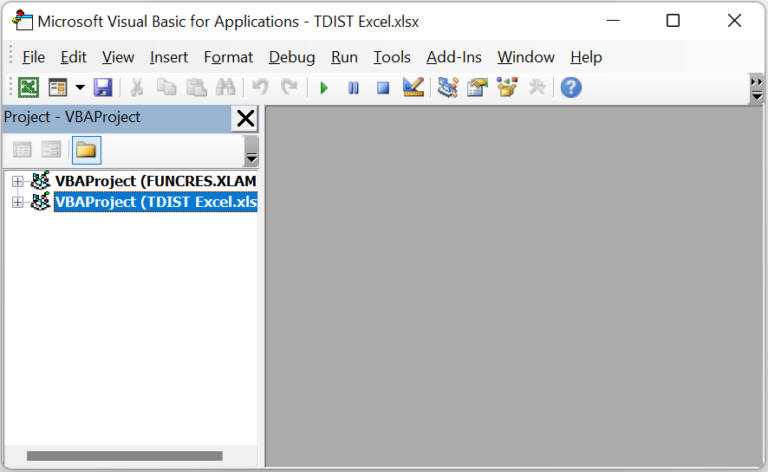• Step 2: Choose the required VBAProject and go to InsertModule to open a new module window, Module1.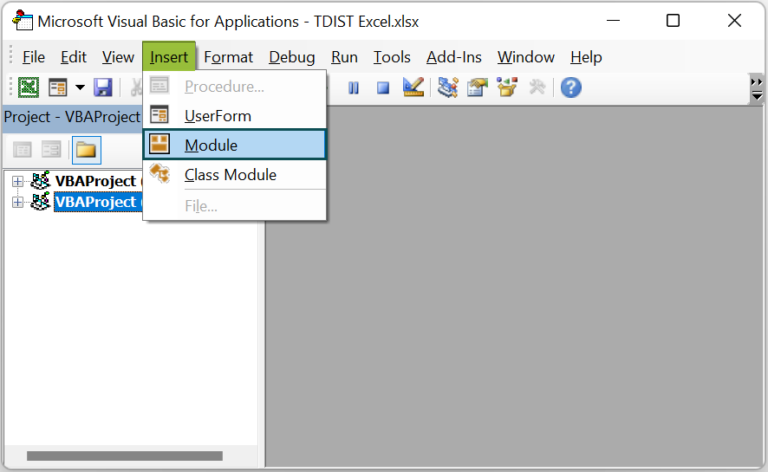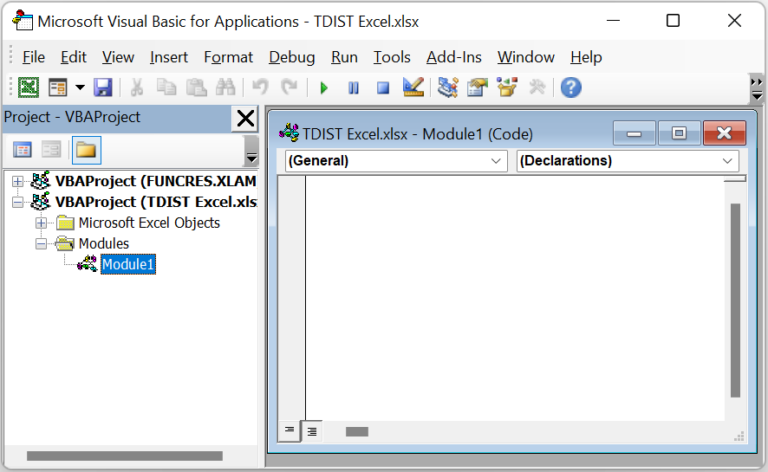• Step 3: Enter the code in the Module1 window to apply the TDIST function in Excel.

Sub TDIST_fn()
Range(“D8”) = Application.worksheetFunction.TDist(Range(“C3”), Range(“C4”), Range(“C5”))
End Sub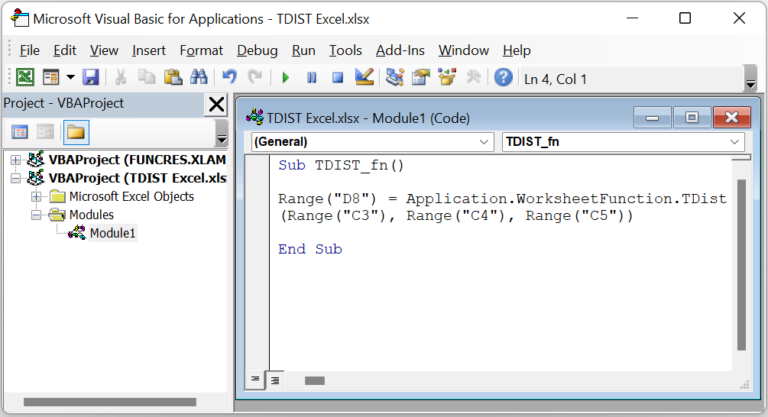• Step 4: Click the Run Sub/UserForm button to run the Module1 code.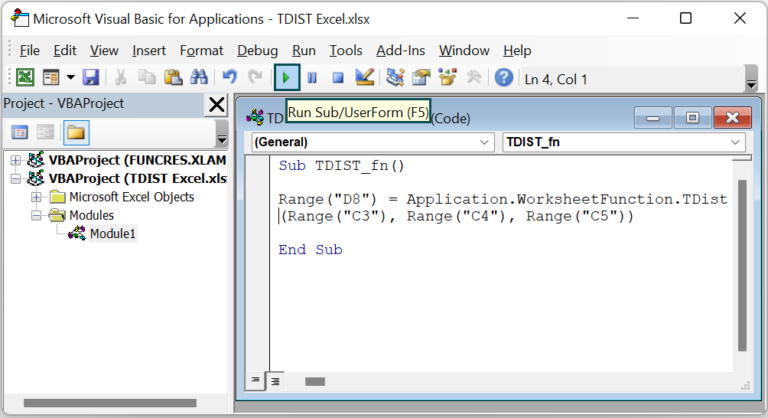And once the code executes, you can view the TDIST() output in the target cell D8.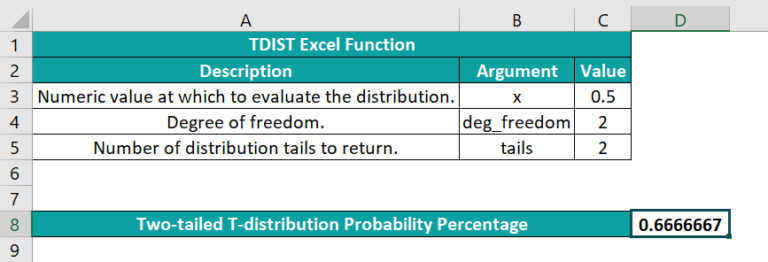• Step 5: Select cell D8 and set the Number Format in the Home tab as Percentage.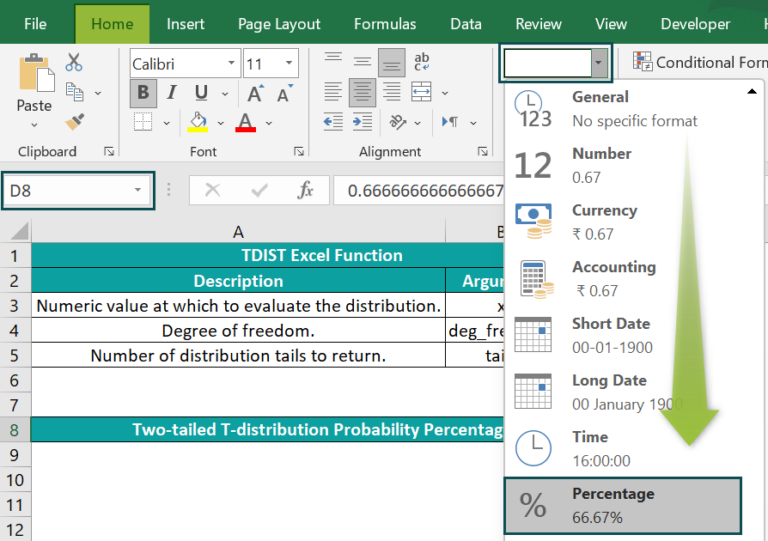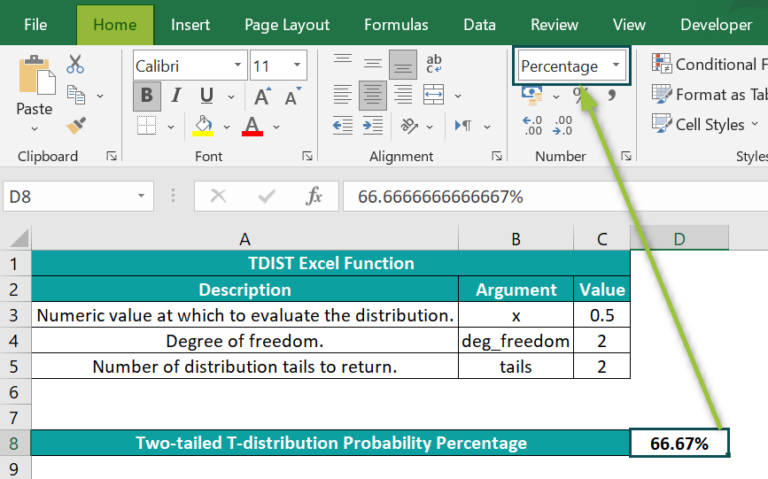The method in the provided code accepts the given input values as the argument values to return the required two-tailed t-distribution probability, 0.6666667.

2. What is the use of the TDIST Excel function?

The use of the TDIST Excel function is that it helps in hypothesis testing of small sample sets.

3. Why is the TDIST function in Excel not working?

The TDIST function in Excel is not working, perhaps because of the following reasons:

You supplied non-numeric argument values to the TDIST().
The provided x value is less than 0.
The supplied deg_freedom argument value is less than 1.
The specified tails argument value is not 1 or 2.